xSeparation of variablesEncyclopedia
In mathematics
Mathematics
Mathematics is the study of quantity, space, structure, and change. Mathematicians seek out patterns and formulate new conjectures. Mathematicians resolve the truth or falsity of conjectures by mathematical proofs, which are arguments sufficient to convince other mathematicians of their validity...

, separation of variables is any of several methods for solving ordinary and partial differential equation
Differential equation
A differential equation is a mathematical equation for an unknown function of one or several variables that relates the values of the function itself and its derivatives of various orders...

s, in which algebra allows one to rewrite an equation so that each of two variables occurs on a different side of the equation.

## Ordinary differential equations (ODE)

Suppose a differential equation can be written in the form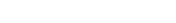which we can write more simply by letting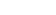: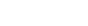As long as h(y) ≠ 0, we can rearrange terms to obtain: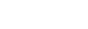so that the two variables x and y have been separated. dx (and dy) can be viewed, at a simple level, as just a convenient notation, which provides a handy mnemonic aid for assisting with manipulations. A formal definition of dx as a differential (infinitesimal) is somewhat advanced.

### Alternative notation

Some who dislike Leibniz's notation may prefer to write this as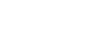but that fails to make it quite as obvious why this is called "separation of variables".

Integrating both sides of the equation with respect to, we have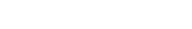or equivalently,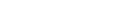because of the substitution rule for integrals
Integration by substitution
In calculus, integration by substitution is a method for finding antiderivatives and integrals. Using the fundamental theorem of calculus often requires finding an antiderivative. For this and other reasons, integration by substitution is an important tool for mathematicians...

.

If one can evaluate the two integrals, one can find a solution to the differential equation. Observe that this process effectively allows us to treat the derivative
Derivative
In calculus, a branch of mathematics, the derivative is a measure of how a function changes as its input changes. Loosely speaking, a derivative can be thought of as how much one quantity is changing in response to changes in some other quantity; for example, the derivative of the position of a...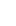as a fraction which can be separated. This allows us to solve separable differential equations more conveniently, as demonstrated in the example below.

(Note that we do not need to use two constants of integration
Arbitrary constant of integration
In calculus, the indefinite integral of a given function is only defined up to an additive constant, the constant of integration. This constant expresses an ambiguity inherent in the construction of antiderivatives...

, in equation (2) as in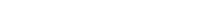because a single constant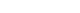is equivalent.)

### Example (I)

The ordinary differential equation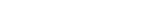may be written as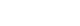If we let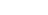and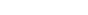, we can write the differential equation in the form of equation (1) above. Thus, the differential equation is separable.

As shown above, we can treat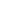and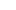as separate values, so that both sides of the equation may be multiplied by. Subsequently dividing both sides by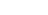, we have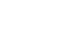At this point we have separated the variables x and y from each other, since x appears only on the right side of the equation and y only on the left.

Integrating both sides, we get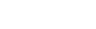which, via partial fraction
Partial fraction
In algebra, the partial fraction decomposition or partial fraction expansion is a procedure used to reduce the degree of either the numerator or the denominator of a rational function ....

s, becomes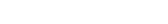and then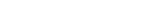where C is the constant of integration
Arbitrary constant of integration
In calculus, the indefinite integral of a given function is only defined up to an additive constant, the constant of integration. This constant expresses an ambiguity inherent in the construction of antiderivatives...

. A bit of algebra
Algebra
Algebra is the branch of mathematics concerning the study of the rules of operations and relations, and the constructions and concepts arising from them, including terms, polynomials, equations and algebraic structures...

gives a solution for y: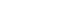One may check our solution by taking the derivative with respect to x of the function we found, where B is an arbitrary constant. The result should be equal to our original problem. (One must be careful with the absolute values when solving the equation above. It turns out that the different signs of the absolute value contribute the positive and negative values for B, respectively. And the B = 0 case is contributed by the case that y = 1, as discussed below.)

Note that since we divided by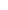and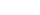we must check to see whether the solutions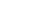and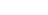solve the differential equation
Differential equation
A differential equation is a mathematical equation for an unknown function of one or several variables that relates the values of the function itself and its derivatives of various orders...

(in this case they are both solutions). See also: singular solution
Singular solution
A singular solution ys of an ordinary differential equation is a solution that is singular or one for which the initial value problem fails to have a unique solution at some point on the solution. The set on which a solution is singular may be as small as a single point or as large as the full...

s.

### Example (II)

Population growth is often modeled by the differential equation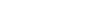where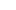is the population with respect to time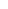,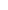is the rate of growth, and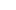is the carrying capacity
Carrying capacity
The carrying capacity of a biological species in an environment is the maximum population size of the species that the environment can sustain indefinitely, given the food, habitat, water and other necessities available in the environment...

of the environment.

Separation of variables may be used to solve this differential equation.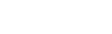To evaluate the integral on the left side, we simplify the fraction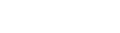and then, we decompose the fraction into partial fractions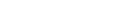Thus we have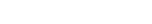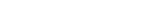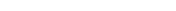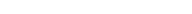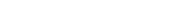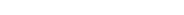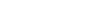Let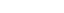.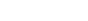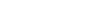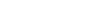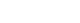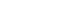Therefore, the solution to the logistic equation is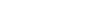To find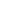, let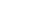and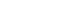. Then we have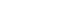Noting that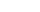, and solving for A we get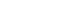## Partial differential equations

The method of separation of variables are also used to solve a wide range of linear partial differential equations with boundary and initial conditions, such as heat equation
Heat equation
The heat equation is an important partial differential equation which describes the distribution of heat in a given region over time...

, wave equation
Wave equation
The wave equation is an important second-order linear partial differential equation for the description of waves – as they occur in physics – such as sound waves, light waves and water waves. It arises in fields like acoustics, electromagnetics, and fluid dynamics...

, Laplace equation and Helmholtz equation
Helmholtz equation
The Helmholtz equation, named for Hermann von Helmholtz, is the elliptic partial differential equation\nabla^2 A + k^2 A = 0where ∇2 is the Laplacian, k is the wavenumber, and A is the amplitude.-Motivation and uses:...

.

### Homogeneous case

Consider the one-dimensional heat equation
Heat equation
The heat equation is an important partial differential equation which describes the distribution of heat in a given region over time...

.The equation is
The boundary condition is homogeneous, that is
Let us attempt to find a solution which is not identically zero satisfying the boundary conditions but with the following property: u is a product in which the dependence of u on x, t is separated, that is:
Substituting u back into equation,
Since the right hand side depends only on x and the left hand side only on t, both sides are equal to some constant value − λ. Thus:
and
− λ here is the eigenvalue for both differential operators, and T(t) and X(x) are corresponding eigenfunction
Eigenfunction
In mathematics, an eigenfunction of a linear operator, A, defined on some function space is any non-zero function f in that space that returns from the operator exactly as is, except for a multiplicative scaling factor. More precisely, one has...

s.

We will now show that solutions for X(x) for values of λ ≤ 0 cannot occur:

Suppose that λ < 0. Then there exist real numbers B, C such that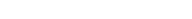From we get
and therefore B = 0 = C which implies u is identically 0.

Suppose that λ = 0. Then there exist real numbers B, C such that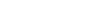From we conclude in the same manner as in 1 that u is identically 0.

Therefore, it must be the case that λ > 0. Then there exist real numbers A, B, C such that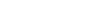and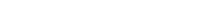From we get C = 0 and that for some positive integer n,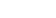This solves the heat equation in the special case that the dependence of u has the special form of .

In general, the sum of solutions to which satisfy the boundary conditions also satisfies and . Hence a complete solution can be given as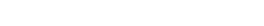where Dn are coefficients determined by initial condition.

Given the initial condition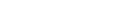we can get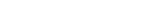This is the sine series expansion of f(x). Multiplying both sides with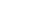and integrating over [0,L] result in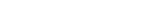This method requires that the eigenfunctions of x, here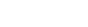, are orthogonal and complete. In general this is guaranteed by Sturm-Liouville theory
Sturm-Liouville theory
In mathematics and its applications, a classical Sturm–Liouville equation, named after Jacques Charles François Sturm and Joseph Liouville , is a real second-order linear differential equation of the form...

.

### Nonhomogeneous case

Suppose the equation is nonhomogeneous,
with the boundary condition the same as .

Expand h(x,t) ,u(x,t) and f(x,t) into
where hn(t) and bn can be calculated by integration, while un(t) is to be determined.

Substitute and back to and considering the orthogonality of sine functions we get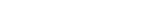which are a sequence of linear differential equations that can be readily solved with, for instance, Laplace transform,or Integrating factor
Integrating factor
In mathematics, an integrating factor is a function that is chosen to facilitate the solving of a given equation involving differentials. It is commonly used to solve ordinary differential equations, but is also used within multivariable calculus, in this case often multiplying through by an...

. Finally, we can get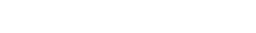If the boundary condition is nonhomogeneous, then the expansion of and is no longer valid. One has to find a function v that satisfies the boundary condition only, and subtract it from u. The function u-v then satisfies homogeneous boundary condition, and can be solved with the above method.

In orthogonal curvilinear coordinates, separation of variables can still be used, but in some details different from that in Cartesian coordinates. For instance, regularity or periodic condition may determine the eigenvalues in place of boundary conditions. See spherical harmonics for example.

## Matrices

The matrix form of the separation of variables is the Kronecker sum.

As an example we consider the 2D discrete Laplacian
Discrete Laplace operator
In mathematics, the discrete Laplace operator is an analog of the continuous Laplace operator, defined so that it has meaning on a graph or a discrete grid...

on a regular grid
Regular grid
A regular grid is a tessellation of n-dimensional Euclidean space by congruent parallelotopes . Grids of this type appear on graph paper and may be used in finite element analysis as well as finite volume methods and finite difference methods...

: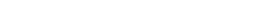where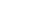and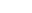are 1D discrete Laplacians in the x- and y-directions, correspondingly, and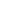are the identities of appropriate sizes. See the main article Kronecker sum of discrete Laplacians
Kronecker sum of discrete Laplacians
In mathematics, the Kronecker sum of discrete Laplacians, named after Leopold Kronecker, is a discrete version of the separation of variables for the continuous Laplacian in a rectangular cuboid domain.-General form of the Kronecker sum of discrete Laplacians:...

for details.

## External links

The source of this article is wikipedia, the free encyclopedia.  The text of this article is licensed under the GFDL.